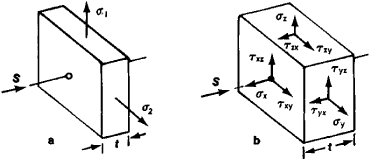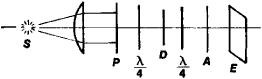# Photoelastic Testing

The following article is from The Great Soviet Encyclopedia (1979). It might be outdated or ideologically biased.

## Photoelastic Testing

a method of studying stresses in machine parts and structural components by the use of transparent models. It is based on the property of most transparent isotropic materials (glass, celluloid, gelatin, and plastics—that is, optically sensitive or piezooptic materials) of becoming optically anisotropic when deformed. In other words, it is based on the appearance of artificial double refraction, or the piezooptic effect.Figure 1. Schematics: (a) a plate loaded in its own plane, (b) a volume element in the stressed state; (σ) normal stresses, (τ) shear stresses

The relationship between the principal values of the dielectric-constant tensor and the principal stresses is linear. Thus, for a plate loaded in its own plane, the principal stress σ3 directed normal to the plate (Figure 1,a) is equal to zero, and one of the principal planes of optical symmetry coincides with the plane of the plate. If light is incident on a plate D in a circular polariscope (Figure 2) perpendicular to the plane of the plate, the optical path difference is equal to Δ = d(n1n2) or Δ = cd1 — σ2), where d is the thickness of the plate, σ1 and σ2 are the principal stresses, and c is the relative stress-optical coefficient. This equation, which is called the Wertheim equation, is of fundamental importance in the solution of two-dimensional photoelasticity problems. When the model is illuminated by monochromatic light, extinction of the light is observed at the points of the model’s interference fringes where Δ = mλ (m is an integer). Maximum light intensity is observed at the points where Δ = (2m + 1)λ/2. Light and dark fringes of different orders m—the fringe pattern—are obtained on the image of the model. Points lying in the same fringe have an identical Δ, that is, identical σ1 — σ2 = 2τmax = Δ/cd, where τmax is the maximum shear stress. When white light is used, points with identical τmax are connected by lines of identical color called isochromatic lines.Figure 2. Schematic of a circular polariscope: (S) light source, (P) polarizer, (D) plate, (λ/4) quarter-wave plates, (A) analyzer, (E) screen

In order to determine σ1 — σ2 or τmax at a given point it is sufficient to determine c for the material of the model and to measure Δ with a compensator. Alternatively, σ0 for the model can be determined, and the order of the fringe m can be calculated. Here, σ0 = λ/cd is the difference between the principal stresses in the model that give the path difference Δ = λ; c and σ0 are obtained by simple extension, compression, or pure bending in samples of the material of which the model is made. When a plane model is illuminated normally, only the difference and direction of the principal stresses can be obtained. For this reason, in order to determine σ1 and σ2 separately, there are used additional physicomechanical techniques for measuring σ1 + σ2 and graphical computational methods for separating σ1 and σ2 on the basis of known σ1 — σ2 and the directions of σ1 and (σ2; the equations of continuum mechanics are used in these methods.

More complex experimental techniques are used to investigate stresses in three-dimensional models. A three-dimensional model is often studied by the method of stress freezing. A model made of a material that has the freezing property—such as hardened epoxy and phenol-formaldehyde resins—is heated to the temperature of the hyperelastic state, loaded, and cooled under load to room temperature (the vitrification temperature). After the load is removed, the stresses that appeared in the hyperelastic state and the accompanying optical anisotropy remain fixed. This phenomenon can be described by means of a conventional two-phase model of the material. When the material is heated to a temperature of 80° to 120°C (the hyperelastic state), one part of the material softens, while the other remains elastic. The load applied to the heated model is resisted by the unsoftened skeleton. When the loaded model is cooled to room temperature, the softening part once again congeals (freezes) and retains the strain in the skeleton after the load is lifted. The frozen model is cut into thin plates, or slices, 0.6 to 2 mm thick, which are studied in an ordinary polariscope.

A scattered light method is also used, wherein a thin beam of parallel rays of polarized light passes through the three-dimensional model and produces at each point of its path scattered light, which is observed in a direction perpendicular to the beam. The state of polarization along the line of each ray changes from point to point according to the stresses at these points. There exists a method in which thin plates of an optically sensitive material are bonded into a three-dimensional model made of a transparent material, such as a special organic glass, that is optically insensitive to stresses. Measurements in the plates are made as in a two-dimensional plane model—with illumination perpendicular or oblique to the surface of the plate.

The photoelastic method of stress determination described above is used to study stresses in flat and three-dimensional parts within the elastic limits in cases where it is difficult or impossible to use computational techniques. The method is used in the investigation of plastic deformations (photoplasticity), dynamic processes, and thermal stresses (photothermoelasticity). It is also used for simulation in solving problems of creep (photocreep) and other nonlinear problems of the mechanics of deformable bodies.

A method involving the application of optically sensitive layers to the surfaces of full-scale parts has also been developed. A layer of optically sensitive material in liquid form is applied to the surface of a metal part or a model thereof; the layer is then subjected to polymerization or is glued to the part in sheet form. This process ensures that the deformations of the loaded part and coating are equal. The strains in the coating are determined from the path difference in the coating measured in reflected light by means of one-way polariscopes.

Since photoelastic testing involves the use of models, the transition from stresses in the model to stresses in the part must be made. In the simplest case σpart = σmodelβ/α2, where α and β are the geometric and force scaling factors, respectively.

### REFERENCES

Prigorovskii, N. I., “Poliarizatsionno-opticheskii metod issledovaniia raspredeleniia napriazhenii.” In Spravochnik mashinostroitelia, vol. 3. Moscow, 1962.
Aleksandrov, A. Ia., and M. Kh. Akhmetzianov. Poliarizatsionno-opticheskie metody mekhaniki deformiruemogo tela. Moscow, 1973.

V. I. SAVCHENKO